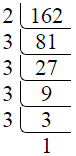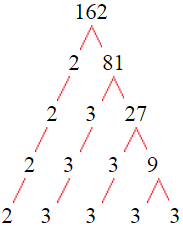# Factors of 162The factors of 162 are 1, 2, 3, 6, 9, 18, 27, 54, 81, and 162 i.e. F162 = {1, 2, 3, 6, 9, 18, 27, 54, 81, 162}. The factors of 162 are all the numbers that can divide 162 without leaving a remainder.

We can check if these numbers are factors of 162 by dividing 162 by each of them. If the result is a whole number, then the number is a factor of 162. Let's do this for each of the numbers listed above:

·        1 is a factor of 162 because 162 divided by 1 is 162.

·        2 is a factor of 162 because 162 divided by 2 is 81.

·        3 is a factor of 162 because 162 divided by 3 is 54.

·        6 is a factor of 162 because 162 divided by 6 is 27.

·        9 is a factor of 162 because 162 divided by 9 is 18.

·        18 is a factor of 162 because 162 divided by 18 is 9.

·        27 is a factor of 162 because 162 divided by 27 is 6.

·        54 is a factor of 162 because 162 divided by 54 is 3.

·        81 is a factor of 162 because 162 divided by 81 is 2.

·        162 is a factor of 162 because 162 divided by 162 is 1.

## How to Find Factors of 162?

1 and the number itself are the factors of every number. So, 1 and 162 are two factors of 162. To find the other factors of 162, we can start by dividing 162 by the numbers between 1 and 162. If we divide 162 by 2, we get a remainder of 0. Therefore, 2 is a factor of 162. If we divide 162 by 3, we get a remainder of 0. Therefore, 3 is also a factor of 162.

Next, we can check if 4 is a factor of 162. If we divide 162 by 4, we get a remainder of 2. Therefore, 4 is not a factor of 162. We can continue this process for all the possible factors of 162.

Through this process, we can find that the factors of 162 are 1, 2, 3, 6, 9, 18, 27, 54, 81, and 162. These are the only numbers that can divide 162 without leaving a remainder.

********************

********************

## Properties of the Factors of 162

The factors of 162 have some interesting properties. One of the properties is that the sum of the factors of 162 is equal to 363. We can see this by adding all the factors of 162 together:

1 + 2 + 3 + 6 + 9 + 18 + 27 + 54 + 81 + 162 = 363

Another property of the factors of 162 is that the prime factors of 162 are 2, and 3 only.

## Applications of the Factors of 162

The factors of 162 have several applications in mathematics. One of the applications is in finding the highest common factor (HCF) of two or more numbers. The HCF is the largest factor that two or more numbers have in common. For example, to find the HCF of 162 and 84, we need to find the factors of both numbers and identify the largest factor they have in common. The factors of 162 are 1, 2, 3, 6, 9, 18, 27, 54, 81, and 162. The factors of 84 are 1, 2, 3, 4, 6, 7, 12, 14, 21, 28, 42, and 84. The largest factor that they have in common is 6. Therefore, the HCF of 162 and 84 is 6.

Another application of the factors of 162 is in prime factorization. Prime factorization is the process of expressing a number as the product of its prime factors. The prime factors of 162 are 2, and 3, since these are the only prime numbers that can divide 162 without leaving a remainder. Therefore, we can express 162 as:

162 = 2 × 3 × 3 × 3 × 3

We can do prime factorization by division and factor tree method also. Here is the prime factorization of 162 by division method,162 = 2 × 3 × 3 × 3 × 3

Here is the prime factorization of 162 by the factor tree method,162 = 2 × 3 × 3 × 3 × 3

## Conclusion

The factors of 162 are the numbers that can divide 162 without leaving a remainder. The factors of 162 are 1, 2, 3, 6, 9, 18, 27, 54, 81, and 162. The factors of 162 have some interesting properties, such as having a sum of 363. The factors of 162 have several applications in mathematics, such as finding the highest common factor and prime factorization.